2020-10-15 13:55:50

## 用20行python代码给证件照换底色

17 / 0 / 0 / 0

#### 1.需求说明声明：该图片来源于百度图片搜索，仅作为技术演示使用。如有侵权，请联系我们删除！

#### 2.读取图片并显示

• imshow()：展示图片；
• waitkey()：设置窗口等待，如果不设置，窗口会一闪而过；
    import cv2
import numpy as np
# 读取照片

# 显示图像
cv2.imshow('img',img)

# 窗口等待的命令，0表示无限等待
cv2.waitKey(0)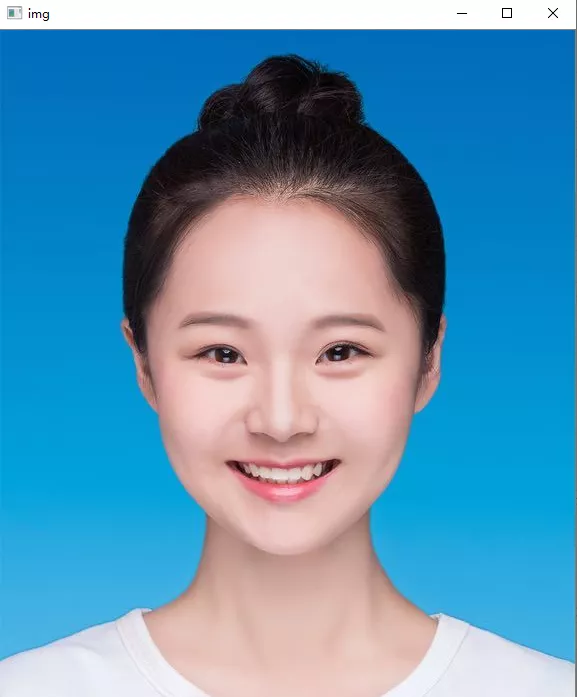#### 3.图片缩放

• resize()：图片缩放，其中fx和fy表示缩放比例，0.5表示缩放为以前的 一半。

import cv2
import numpy as np
# 读取照片

# 图像缩放
img = cv2.resize(img,None,fx=0.5,fy=0.5)
rows,cols,channels = img.shape
print(rows,cols,channels)

# 显示图像
cv2.imshow('img',img)

# 窗口等待的命令，0表示无限等待
cv2.waitKey(0)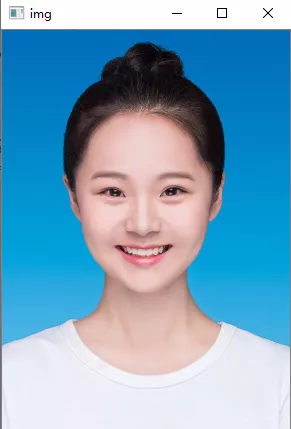#### 4.将图片转换为灰度图像

• cv2.cvtColor(img,cv2.COLOR_BGR2HSV)可以将彩色图片转化为hsv灰度图片。
    import cv2
import numpy as np
# 读取照片

# 图像缩放
img = cv2.resize(img,None,fx=0.5,fy=0.5)
rows,cols,channels = img.shape
print(rows,cols,channels)
cv2.imshow('img',img)

# 图片转换为二值化图
hsv = cv2.cvtColor(img,cv2.COLOR_BGR2HSV)

# 显示图像
cv2.imshow('hsv',hsv)

# 窗口等待的命令，0表示无限等待
cv2.waitKey(0)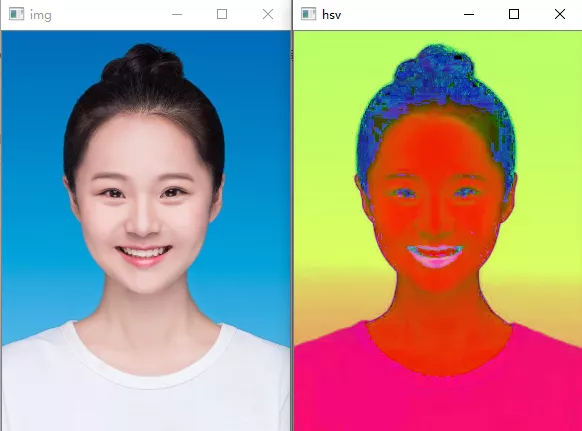#### 5.将图片进行二值化处理

• lower_blue = np.array([90,70,70])
• upper_blue = np.array([110,255,255])
• inRange(hsv, lower_blue, upper_blue)将图片进行二值化操作。
    import cv2
import numpy as np
# 读取照片

# 图像缩放
img = cv2.resize(img,None,fx=0.5,fy=0.5)
rows,cols,channels = img.shape
print(rows,cols,channels)
cv2.imshow('img',img)

# 图片转换为灰度图
hsv = cv2.cvtColor(img,cv2.COLOR_BGR2HSV)
cv2.imshow('hsv',hsv)

# 图片的二值化处理
lower_blue = np.array([90,70,70])
upper_blue = np.array([110,255,255])

# 显示图像

# 窗口等待的命令，0表示无限等待
cv2.waitKey(0)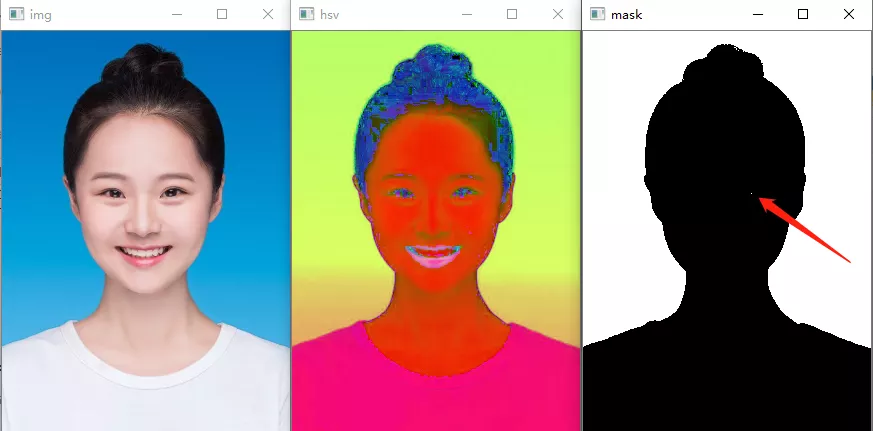#### 6.图象的腐蚀和膨胀

• dilate(erode,None,iterations=1)进行膨胀操作。
    import cv2
import numpy as np
# 读取照片

# 图像缩放
img = cv2.resize(img,None,fx=0.5,fy=0.5)
rows,cols,channels = img.shape
print(rows,cols,channels)
cv2.imshow('img',img)

# 图片转换为灰度图
hsv = cv2.cvtColor(img,cv2.COLOR_BGR2HSV)
cv2.imshow('hsv',hsv)

# 图片的二值化处理
lower_blue=np.array([90,70,70])
upper_blue=np.array([110,255,255])

#腐蚀膨胀
cv2.imshow('erode',erode)

dilate=cv2.dilate(erode,None,iterations=1)
cv2.imshow('dilate',dilate)

# 窗口等待的命令，0表示无限等待
cv2.waitKey(0)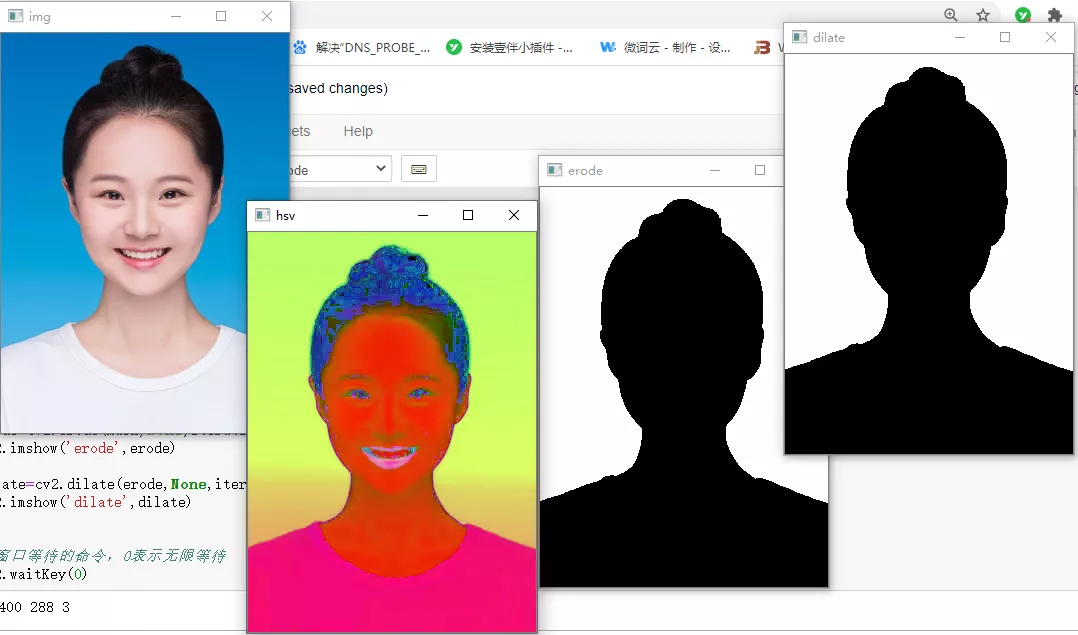#### 7.遍历每个像素点进行颜色替换

import cv2
import numpy as np
# 读取照片

# 图像缩放
img = cv2.resize(img,None,fx=0.5,fy=0.5)
rows,cols,channels = img.shape
print(rows,cols,channels)
cv2.imshow('img',img)

# 图片转换为灰度图
hsv = cv2.cvtColor(img,cv2.COLOR_BGR2HSV)
cv2.imshow('hsv',hsv)

# 图片的二值化处理
lower_blue=np.array([90,70,70])
upper_blue=np.array([110,255,255])

#腐蚀膨胀
cv2.imshow('erode',erode)

dilate=cv2.dilate(erode,None,iterations=1)
cv2.imshow('dilate',dilate)

#遍历每个像素点，进行颜色的替换
for i in range(rows):
for j in range(cols):
if erode[i,j]==255: # 像素点为255表示的是白色，我们就是要将白色处的像素点，替换为红色
img[i,j]=(0,0,255) # 此处替换颜色，为BGR通道，不是RGB通道
cv2.imshow('res',img)

# 窗口等待的命令，0表示无限等待
cv2.waitKey(0)  

PS: 如本文对您有疑惑，可加QQ：1752338621 进行讨论。

0
0